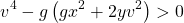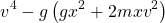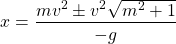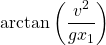# Change FastCast's projectile acceleration to achieve parabolic trajectory

Hello I am using the FastCast API to manage projectiles in my tool wrapper module and I wanted to ask how could I change the acceleration of the projectile depending on the distance from the position I aimed at to make it hit/destroy itself there (like in the picture) with a parabolic trajectory (like basketball)

This is the code I use for firing function (some parts are from the example gun):

``````		-- Shortcuts
local pointerRaycastResult = inputData.RaycastResult
local projectile = self.Config.Projectile
-- Shortcuts

for i = 1, projectile.PerShot do
-- If the input raycast didn't hit anything (likely when there was no object in front of the raycast)
-- set the raycast hit position to farthest it could possibly go
local raycastHitPosition = if pointerRaycastResult then pointerRaycastResult.Position else inputData.FarthestRaycastPosition

-- Direction to the raycast hit position relative to tool's fire point position
local inputDirection = (raycastHitPosition - self.FirePoint.WorldPosition).Unit

local orientational = CFrame.fromOrientation(0, 0, Randomizer:NextNumber(0, Tau))
local directional = CFrame.new(Vector3.new(), inputDirection)

local direction = (directional * orientational * angleSpread).LookVector

local distance = (self.FirePoint.WorldPosition - raycastHitPosition).Magnitude

local calculatedAcceleration = self.Config.Projectile.Acceleration
self.CastBehavior.Acceleration = calculatedAcceleration

local simBullet = self.Caster:Fire(self.FirePoint.WorldPosition, direction, (direction * projectile.Speed), self.CastBehavior)
end
``````
1 Like

The acceleration should always be the same no matter what the distance is. Acceleration means "how quickly the speed changes.

Seems to me like you just have to do

``````self.CastBehavior.Acceleration = Vector3.new(0, -196.2, 0)
``````
1 Like

Well, the projectile still doesn’t land where I aimed at when I set it to the workspace gravity

Oh sorry, so it seems what you’re asking is how to change the launch angle of the projectile so that, with your acceleration, it lands at the right spot?

1 Like

Yes, sorry, english is not my main language, I didn’t know how to word it properly1 Like

Here’s an example of that equation translated to Lua and moved to 3D. I put this in a LocalScript in StarterCharacterScripts for testing.

It just launches a part by setting it’s `Velocity` and assuming the acceleration is `-workspace.Gravity`, but the `LaunchDirection` code should be easy to adapt to your FastCast code.

You can see how there are two solutions, a higher arc and a lower arc. I toggle between them here, but you could just pick one.

There are some unsolved problems (like if you are too far away to make it with that launch angle, what should you do?) but it’s a good starting point.

Results:

``````local character = script.Parent
local root = character:WaitForChild("HumanoidRootPart")
local mouse = game.Players.LocalPlayer:GetMouse()

-- Compute 2D launch angle
-- v: launch velocity
-- g: gravity (positive) e.g. 196.2
-- d: horizontal distance
-- h: vertical distance
-- higherArc: if true, use the higher arc. If false, use the lower arc.
local function LaunchAngle(v: number, g: number, d: number, h: number, higherArc: boolean)
local v2 = v * v
local v4 = v2 * v2
local root = math.sqrt(v4 - g*(g*d*d + 2*h*v2))
if not higherArc then root = -root end
return math.atan((v2 + root) / (g * d))
end

-- Compute 3D launch direction from
-- start: start position
-- target: target position
-- v: launch velocity
-- g: gravity (positive) e.g. 196.2
-- higherArc: if true, use the higher arc. If false, use the lower arc.
local function LaunchDirection(start, target, v, g, higherArc: boolean)
-- get the direction flattened:
local horizontal = Vector3.new(target.X - start.X, 0, target.Z - start.Z)

local h = target.Y - start.Y
local d = horizontal.Magnitude
local a = LaunchAngle(v, g, d, h, higherArc)

-- NaN ~= NaN, computation couldn't be done (e.g. because it's too far to launch)
if a ~= a then return nil end

-- speed if we were just launching at a flat angle:
local vec = horizontal.Unit * v

-- rotate around the axis perpendicular to that direction...
local rotAxis = Vector3.new(-horizontal.Z, 0, horizontal.X)

-- ...by the angle amount
return CFrame.fromAxisAngle(rotAxis, a) * vec
end

higher = true
mouse.Button1Down:Connect(function()
local dir = LaunchDirection(root.Position, mouse.Hit.Position, 50, workspace.Gravity, higher)
higher = not higher -- toggle
if not dir then
print("nope!")
return
end
local p = Instance.new("Part")
p.Size = Vector3.new(1, 1, 1)
p.CanCollide = false
p.Position = root.Position
p.Velocity = dir
p.Parent = workspace
end)
``````
4 Likes

Here’s some more detail on what to do if the distance is too far. This isn’t really part of your question, but I’ll go into it for posterityHere’s an updated desmos with the max range stuff: Projectile Motion Calculation With Max

Explanation:

A bit of math to help.

The target is only within range when the part under the square root is positive, i.e.We’re trying to solve for `x` here. But we have another unknown, `y` (which equates to “the height of the projectile when we launch it as far as we can”).

Instead, I will calculate the slope of the start → target line (`m = y0/x0`), then replace the `y` with `mx`.

So we’re finding the roots of this quadratic now:Solving using the quadratic formula, simplifying, etc.:This is “the furthest horizontal distance we can get along the start → target slope”.

Now we just need the launch angle to get to that distance:(the same formula as we had originally, but we don’t need the square root because it’s 0)

Turning this into lua:

``````local character = script.Parent
local root = character:WaitForChild("HumanoidRootPart")
local mouse = game.Players.LocalPlayer:GetMouse()

local function MaxDistance(v, g, d, h)
local m = h / d
local v2 = v * v
return (v2*math.sqrt(m*m + 1) - m*v2) / g
end

-- Compute 2D launch angle. First return value is true if within range, second is the angle.
-- v: launch velocity
-- g: gravity (positive) e.g. 196.2
-- d: horizontal distance
-- h: vertical distance
-- higherArc: if true, use the higher arc. If false, use the lower arc.
local function LaunchAngle(v: number, g: number, d: number, h: number, higherArc: boolean): (boolean, number)
local max_x = MaxDistance(v, g, d, h)
local v2 = v * v

if d > max_x then
return false, math.atan(v2 / (g * max_x))
end

local v4 = v2 * v2
local root = math.sqrt(v4 - g*(g*d*d + 2*h*v2))
if not higherArc then root = -root end
return true, math.atan((v2 + root) / (g * d))
end

-- Compute 3D launch direction from. First return value is true if within range, second is the direction.
-- start: start position
-- target: target position
-- v: launch velocity
-- g: gravity (positive) e.g. 196.2
-- higherArc: if true, use the higher arc. If false, use the lower arc.
local function LaunchDirection(start, target, v, g, higherArc: boolean)
-- get the direction flattened:
local horizontal = Vector3.new(target.X - start.X, 0, target.Z - start.Z)

local h = target.Y - start.Y
local d = horizontal.Magnitude
local inRange, a = LaunchAngle(v, g, d, h, higherArc)

-- speed if we were just launching at a flat angle:
local vec = horizontal.Unit * v

-- rotate around the axis perpendicular to that direction...
local rotAxis = Vector3.new(-horizontal.Z, 0, horizontal.X)

-- ...by the angle amount
return inRange, CFrame.fromAxisAngle(rotAxis, a) * vec
end

higher = true
mouse.Button1Down:Connect(function()
local inRange, dir = LaunchDirection(root.Position, mouse.Hit.Position, 50, workspace.Gravity, higher)
higher = not higher -- toggle
local p = Instance.new("Part")
p.Size = Vector3.new(1, 1, 1)
p.CanCollide = false
p.BrickColor = inRange and BrickColor.Green() or BrickColor.Red()
p.Position = root.Position
p.Velocity = dir
p.Parent = workspace
end)
``````
8 Likes

Bro, you really are a math genius, thank you and have a nice new year! Btw how could I make the “higher” angle a bit flatter?

• Just use the lower arc (by passing `false` as the last argument, or leaving it out)
• Decrease the launch speed
• Increase gravity (or your bullet’s downwards acceleration)

Just so you know, this isn’t the only way to solve this problem. For example, you can fix the angle and determine the launch velocity.

You could also have some combination of the two ways depending on what exactly you want the result to look like.

A simple alternative is to calculate the launch angle for like three or four possible launch velocities, and pick the slowest one that works.

1 Like

Oh I got the `higherArc` argument backwards. Replace this line:

``````	if higherArc then root = -root end
``````

with

``````	if not higherArc then root = -root end
``````
1 Like